# Using Transformations to Graph 2 1 Quadratic Functions

• Slides: 41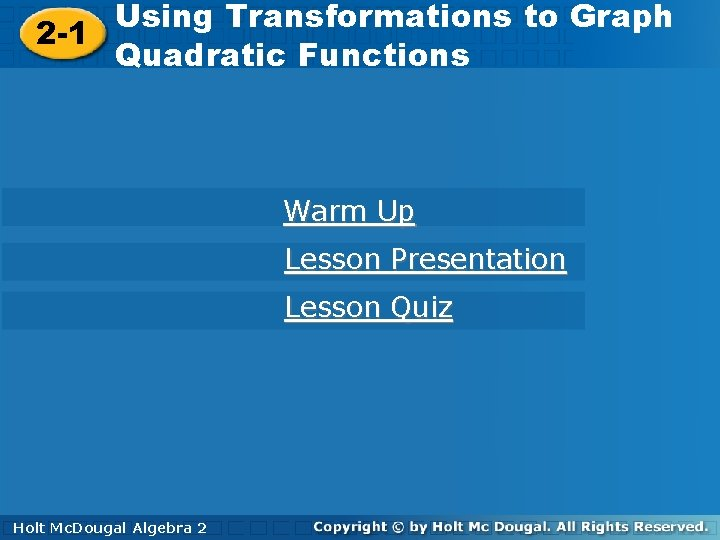Using Transformations to Graph 2 -1 Quadratic Functions Warm Up Lesson Presentation Lesson Quiz Holt. Mc. Dougal Algebra 2 Holt2 -1 Using Transformations to Graph Quadratic Functions Warm Up For each translation of the point (– 2, 5), give the coordinates of the translated point. 1. 6 units down (– 2, – 1) 2. 3 units right (1, 5) For each function, evaluate f(– 2), f(0), and f(3). 3. f(x) = x 2 + 2 x + 6 6; 6; 21 4. f(x) = 2 x 2 – 5 x + 1 19; 1; 4 Holt Mc. Dougal Algebra 22 -1 Using Transformations to Graph Quadratic Functions Objectives Transform quadratic functions. Describe the effects of changes in the coefficients of y = a(x – h)2 + k. Holt Mc. Dougal Algebra 22 -1 Using Transformations to Graph Quadratic Functions Vocabulary quadratic function parabola vertex of a parabola vertex form Holt Mc. Dougal Algebra 2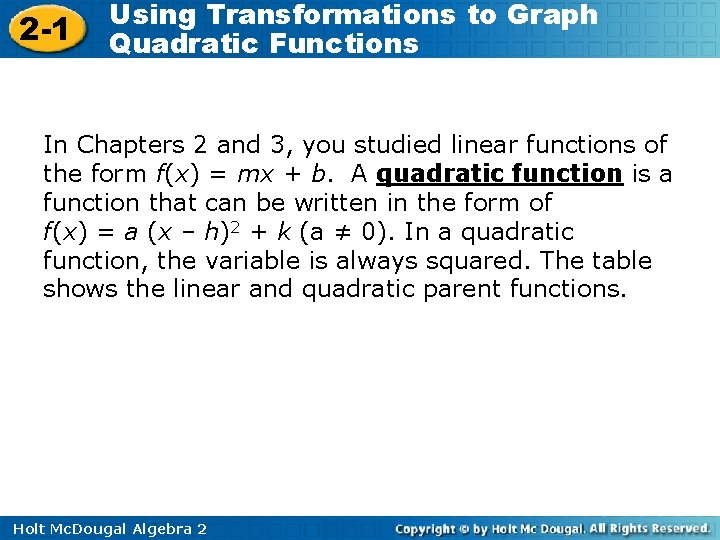2 -1 Using Transformations to Graph Quadratic Functions In Chapters 2 and 3, you studied linear functions of the form f(x) = mx + b. A quadratic function is a function that can be written in the form of f(x) = a (x – h)2 + k (a ≠ 0). In a quadratic function, the variable is always squared. The table shows the linear and quadratic parent functions. Holt Mc. Dougal Algebra 2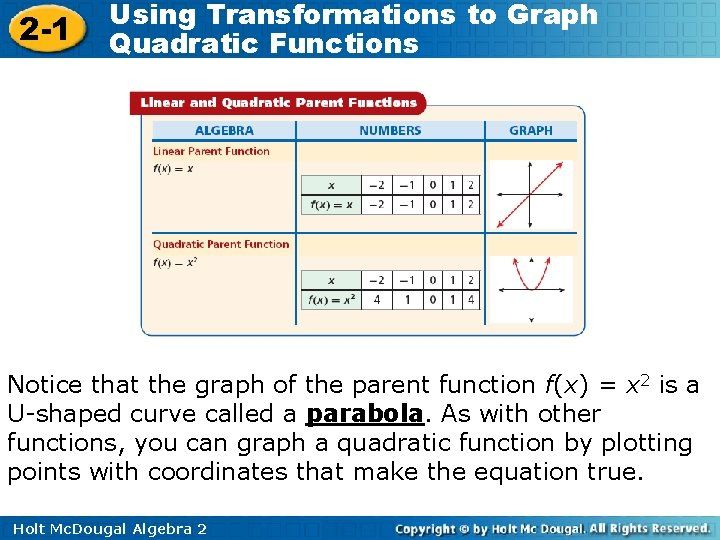2 -1 Using Transformations to Graph Quadratic Functions Notice that the graph of the parent function f(x) = x 2 is a U-shaped curve called a parabola. As with other functions, you can graph a quadratic function by plotting points with coordinates that make the equation true. Holt Mc. Dougal Algebra 2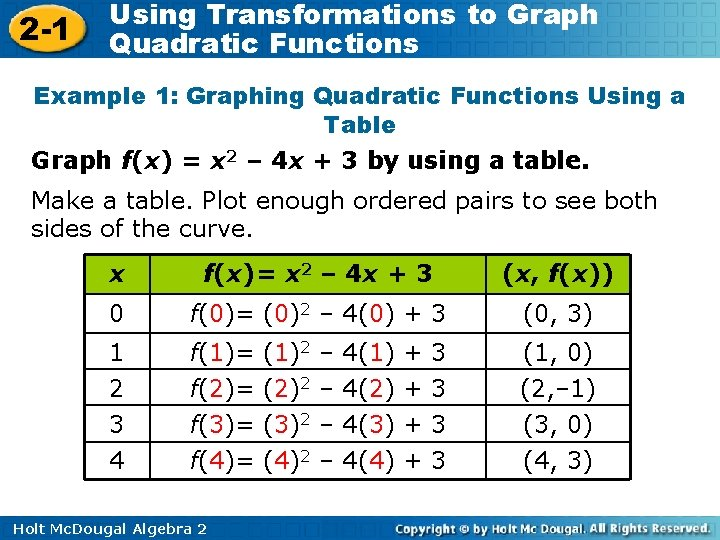2 -1 Using Transformations to Graph Quadratic Functions Example 1: Graphing Quadratic Functions Using a Table Graph f(x) = x 2 – 4 x + 3 by using a table. Make a table. Plot enough ordered pairs to see both sides of the curve. x f(x)= x 2 – 4 x + 3 (x, f(x)) 0 f(0)= (0)2 – 4(0) + 3 (0, 3) 1 2 f(1)= (1)2 – 4(1) + 3 f(2)= (2)2 – 4(2) + 3 (1, 0) (2, – 1) 3 f(3)= (3)2 – 4(3) + 3 (3, 0) 4 f(4)= (4)2 – 4(4) + 3 (4, 3) Holt Mc. Dougal Algebra 22 -1 Using Transformations to Graph Quadratic Functions Example 1 Continued f(x) = x 2 – 4 x + 3 • • • Holt Mc. Dougal Algebra 2 • •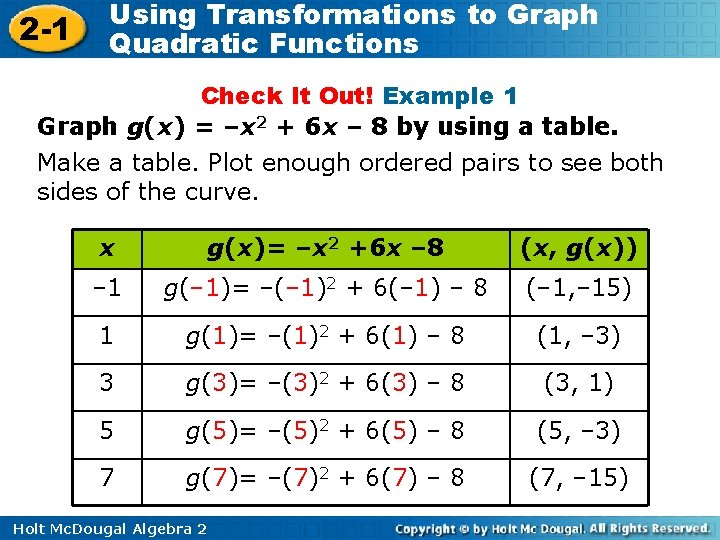2 -1 Using Transformations to Graph Quadratic Functions Check It Out! Example 1 Graph g(x) = –x 2 + 6 x – 8 by using a table. Make a table. Plot enough ordered pairs to see both sides of the curve. x g(x)= –x 2 +6 x – 8 (x, g(x)) – 1 g(– 1)= –(– 1)2 + 6(– 1) – 8 (– 1, – 15) 1 g(1)= –(1)2 + 6(1) – 8 (1, – 3) 3 g(3)= –(3)2 + 6(3) – 8 (3, 1) 5 g(5)= –(5)2 + 6(5) – 8 (5, – 3) 7 g(7)= –(7)2 + 6(7) – 8 (7, – 15) Holt Mc. Dougal Algebra 2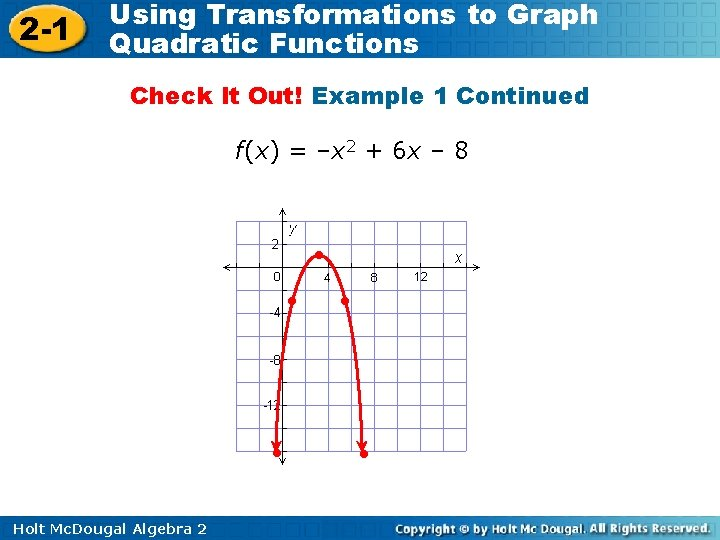2 -1 Using Transformations to Graph Quadratic Functions Check It Out! Example 1 Continued f(x) = –x 2 + 6 x – 8 • • • Holt Mc. Dougal Algebra 2 • •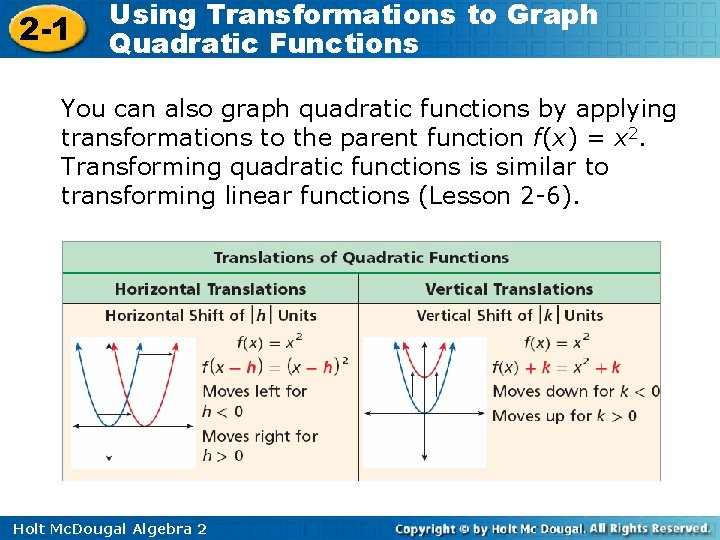2 -1 Using Transformations to Graph Quadratic Functions You can also graph quadratic functions by applying transformations to the parent function f(x) = x 2. Transforming quadratic functions is similar to transforming linear functions (Lesson 2 -6). Holt Mc. Dougal Algebra 22 -1 Using Transformations to Graph Quadratic Functions Example 2 A: Translating Quadratic Functions Use the graph of f(x) = x 2 as a guide, describe the transformations and then graph each function. g(x) = (x – 2)2 + 4 Identify h and k. g(x) = (x – 2)2 + 4 h k Because h = 2, the graph is translated 2 units right. Because k = 4, the graph is translated 4 units up. Therefore, g is f translated 2 units right and 4 units up. Holt Mc. Dougal Algebra 22 -1 Using Transformations to Graph Quadratic Functions Example 2 B: Translating Quadratic Functions Use the graph of f(x) = x 2 as a guide, describe the transformations and then graph each function. g(x) = (x + 2)2 – 3 Identify h and k. g(x) = (x – (– 2))2 + (– 3) h k Because h = – 2, the graph is translated 2 units left. Because k = – 3, the graph is translated 3 units down. Therefore, g is f translated 2 units left and 4 units down. Holt Mc. Dougal Algebra 2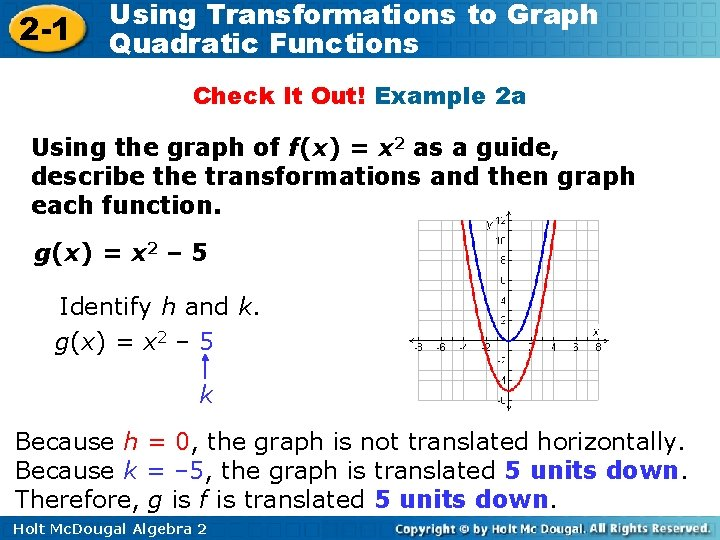2 -1 Using Transformations to Graph Quadratic Functions Check It Out! Example 2 a Using the graph of f(x) = x 2 as a guide, describe the transformations and then graph each function. g(x) = x 2 – 5 Identify h and k. g(x) = x 2 – 5 k Because h = 0, the graph is not translated horizontally. Because k = – 5, the graph is translated 5 units down. Therefore, g is f is translated 5 units down. Holt Mc. Dougal Algebra 2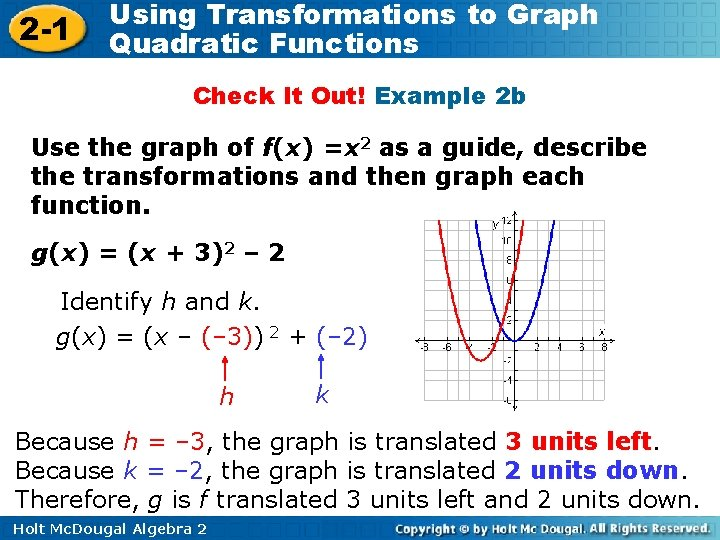2 -1 Using Transformations to Graph Quadratic Functions Check It Out! Example 2 b Use the graph of f(x) =x 2 as a guide, describe the transformations and then graph each function. g(x) = (x + 3)2 – 2 Identify h and k. g(x) = (x – (– 3)) 2 + (– 2) h k Because h = – 3, the graph is translated 3 units left. Because k = – 2, the graph is translated 2 units down. Therefore, g is f translated 3 units left and 2 units down. Holt Mc. Dougal Algebra 22 -1 Using Transformations to Graph Quadratic Functions Recall that functions can also be reflected, stretched, or compressed. Holt Mc. Dougal Algebra 2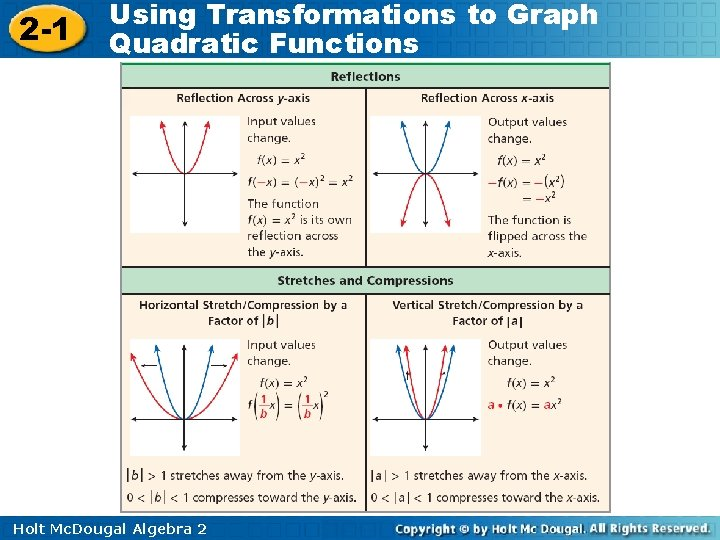2 -1 Using Transformations to Graph Quadratic Functions Holt Mc. Dougal Algebra 22 -1 Using Transformations to Graph Quadratic Functions Example 3 A: Reflecting, Stretching, and Compressing Quadratic Functions Using the graph of f(x) = x 2 as a guide, describe the transformations and then graph each function. g (x ) =- 1 x 4 2 Because a is negative, g is a reflection of f across the x-axis. Because |a| = , g is a vertical compression of f by a factor of. Holt Mc. Dougal Algebra 2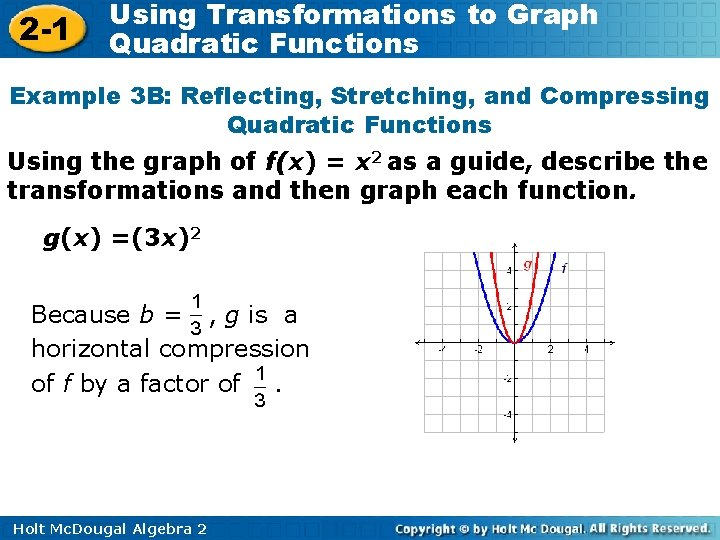2 -1 Using Transformations to Graph Quadratic Functions Example 3 B: Reflecting, Stretching, and Compressing Quadratic Functions Using the graph of f(x) = x 2 as a guide, describe the transformations and then graph each function. g(x) =(3 x)2 Because b = , g is a horizontal compression of f by a factor of. Holt Mc. Dougal Algebra 22 -1 Using Transformations to Graph Quadratic Functions Check It Out! Example 3 a Using the graph of f(x) = x 2 as a guide, describe the transformations and then graph each function. g(x) =(2 x)2 Because b = , g is a horizontal compression of f by a factor of. Holt Mc. Dougal Algebra 22 -1 Using Transformations to Graph Quadratic Functions Check It Out! Example 3 b Using the graph of f(x) = x 2 as a guide, describe the transformations and then graph each function. g(x) = – x 2 Because a is negative, g is a reflection of f across the x-axis. Because |a| = , g is a vertical compression of f by a factor of. Holt Mc. Dougal Algebra 2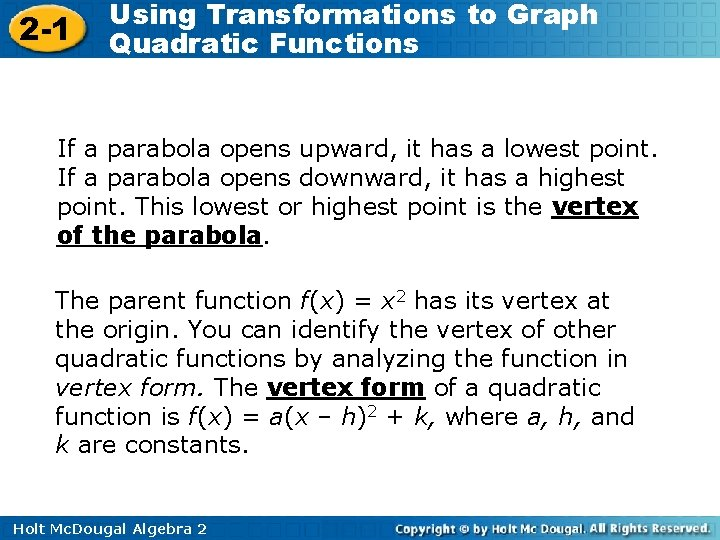2 -1 Using Transformations to Graph Quadratic Functions If a parabola opens upward, it has a lowest point. If a parabola opens downward, it has a highest point. This lowest or highest point is the vertex of the parabola. The parent function f(x) = x 2 has its vertex at the origin. You can identify the vertex of other quadratic functions by analyzing the function in vertex form. The vertex form of a quadratic function is f(x) = a(x – h)2 + k, where a, h, and k are constants. Holt Mc. Dougal Algebra 22 -1 Using Transformations to Graph Quadratic Functions Because the vertex is translated h horizontal units and k vertical from the origin, the vertex of the parabola is at (h, k). Helpful Hint When the quadratic parent function f(x) = x 2 is written in vertex form, y = a(x – h)2 + k, a = 1, h = 0, and k = 0. Holt Mc. Dougal Algebra 2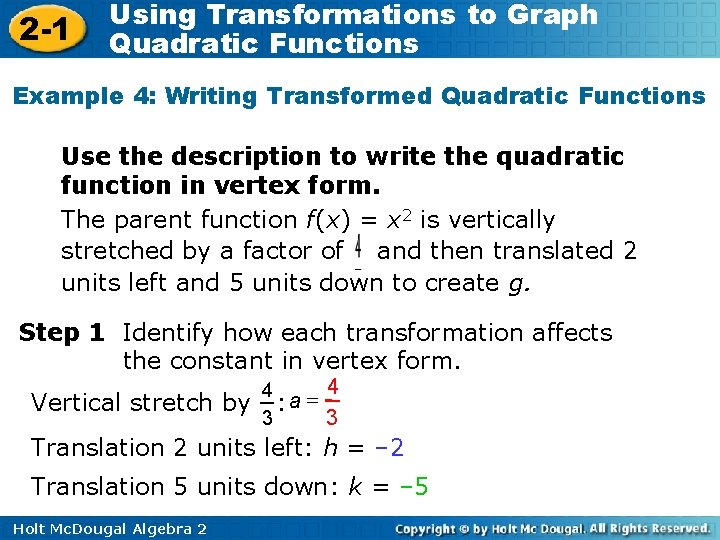2 -1 Using Transformations to Graph Quadratic Functions Example 4: Writing Transformed Quadratic Functions Use the description to write the quadratic function in vertex form. The parent function f(x) = x 2 is vertically stretched by a factor of and then translated 2 units left and 5 units down to create g. Step 1 Identify how each transformation affects the constant in vertex form. Vertical stretch by 4 4 = a : 3 3 Translation 2 units left: h = – 2 Translation 5 units down: k = – 5 Holt Mc. Dougal Algebra 22 -1 Using Transformations to Graph Quadratic Functions Example 4: Writing Transformed Quadratic Functions Step 2 Write the transformed function. g(x) = a(x – h)2 + k Vertex form of a quadratic function = (x – (– 2))2 + (– 5) Substitute = (x + 2)2 – 5 g(x) = (x + 2)2 – 5 Holt Mc. Dougal Algebra 2 Simplify. for a, – 2 for h, and – 5 for k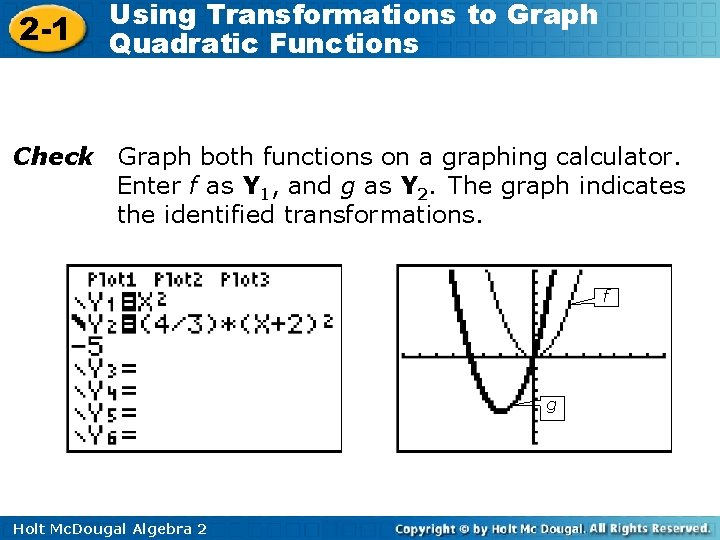2 -1 Check Using Transformations to Graph Quadratic Functions Graph both functions on a graphing calculator. Enter f as Y 1, and g as Y 2. The graph indicates the identified transformations. f g Holt Mc. Dougal Algebra 2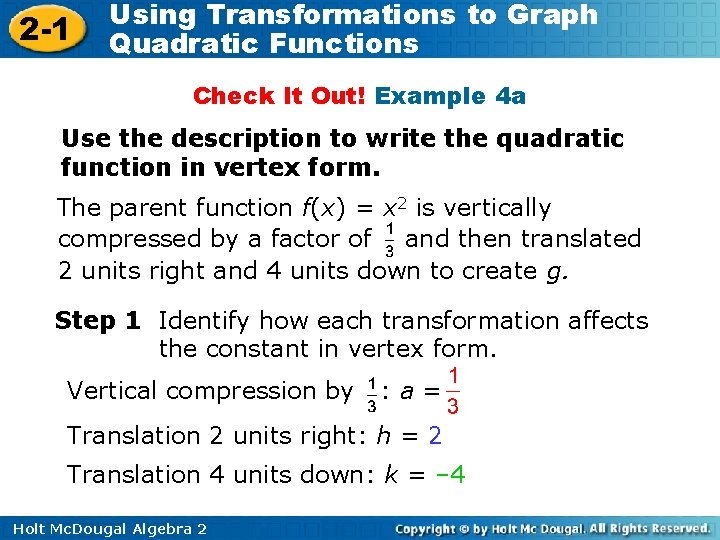2 -1 Using Transformations to Graph Quadratic Functions Check It Out! Example 4 a Use the description to write the quadratic function in vertex form. The parent function f(x) = x 2 is vertically compressed by a factor of and then translated 2 units right and 4 units down to create g. Step 1 Identify how each transformation affects the constant in vertex form. Vertical compression by : a= Translation 2 units right: h = 2 Translation 4 units down: k = – 4 Holt Mc. Dougal Algebra 2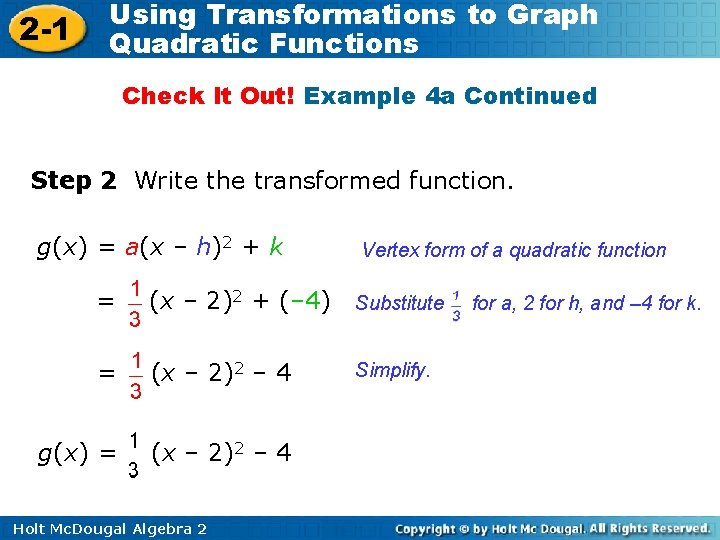2 -1 Using Transformations to Graph Quadratic Functions Check It Out! Example 4 a Continued Step 2 Write the transformed function. g(x) = a(x – h)2 + k Vertex form of a quadratic function = (x – 2)2 + (– 4) Substitute = (x – 2)2 – 4 g(x) = (x – 2)2 – 4 Holt Mc. Dougal Algebra 2 Simplify. for a, 2 for h, and – 4 for k.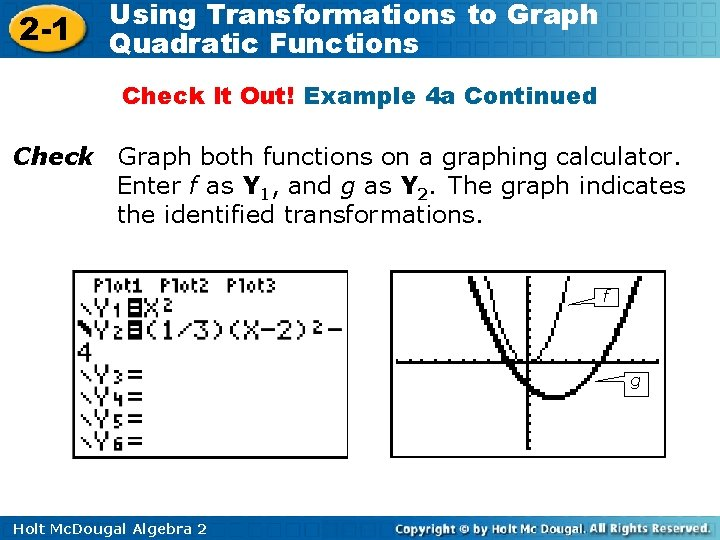2 -1 Using Transformations to Graph Quadratic Functions Check It Out! Example 4 a Continued Check Graph both functions on a graphing calculator. Enter f as Y 1, and g as Y 2. The graph indicates the identified transformations. f g Holt Mc. Dougal Algebra 2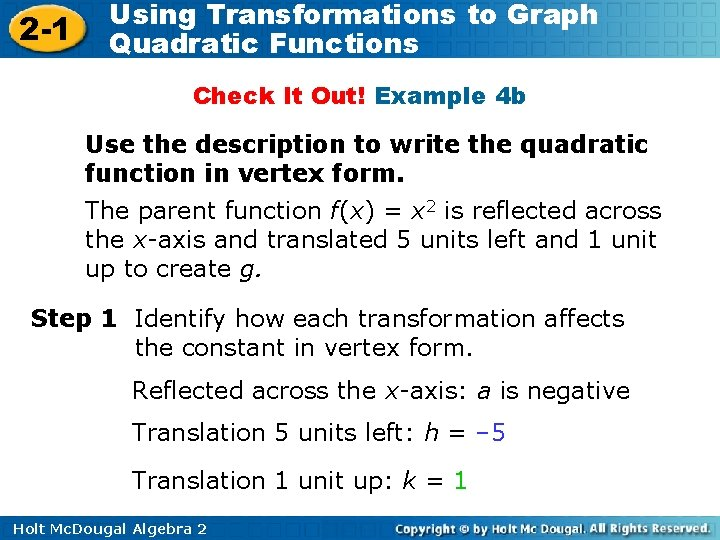2 -1 Using Transformations to Graph Quadratic Functions Check It Out! Example 4 b Use the description to write the quadratic function in vertex form. The parent function f(x) = x 2 is reflected across the x-axis and translated 5 units left and 1 unit up to create g. Step 1 Identify how each transformation affects the constant in vertex form. Reflected across the x-axis: a is negative Translation 5 units left: h = – 5 Translation 1 unit up: k = 1 Holt Mc. Dougal Algebra 2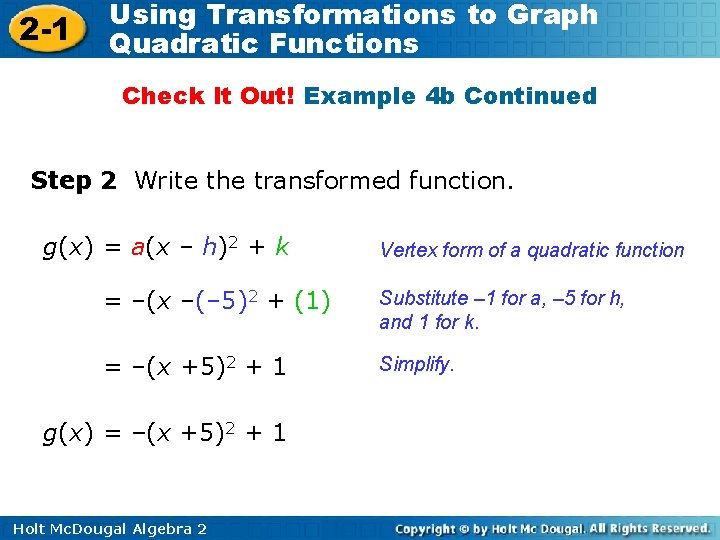2 -1 Using Transformations to Graph Quadratic Functions Check It Out! Example 4 b Continued Step 2 Write the transformed function. g(x) = a(x – h)2 + k Vertex form of a quadratic function = –(x –(– 5)2 + (1) Substitute – 1 for a, – 5 for h, and 1 for k. = –(x +5)2 + 1 Simplify. g(x) = –(x +5)2 + 1 Holt Mc. Dougal Algebra 2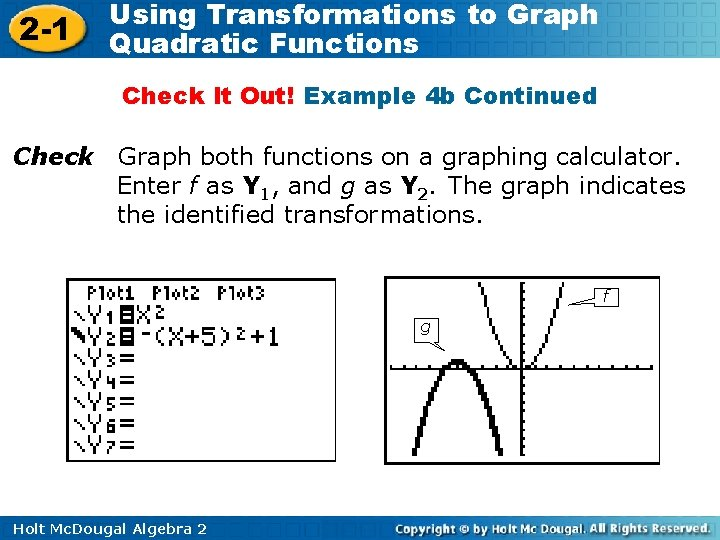2 -1 Using Transformations to Graph Quadratic Functions Check It Out! Example 4 b Continued Check Graph both functions on a graphing calculator. Enter f as Y 1, and g as Y 2. The graph indicates the identified transformations. f g Holt Mc. Dougal Algebra 2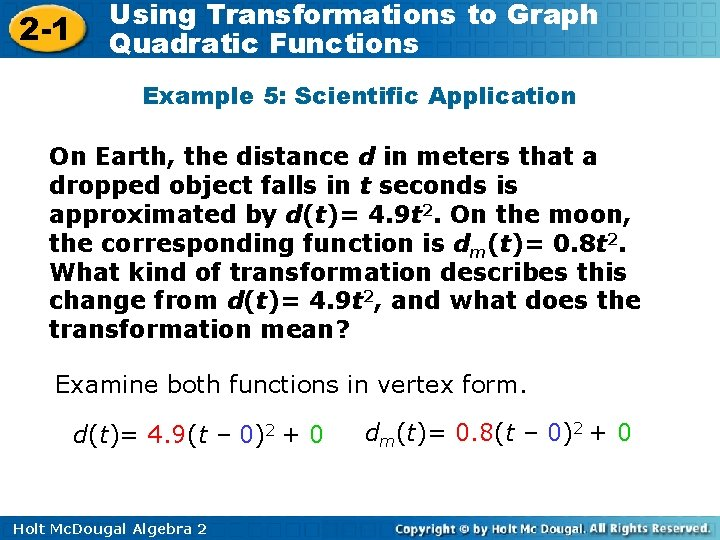2 -1 Using Transformations to Graph Quadratic Functions Example 5: Scientific Application On Earth, the distance d in meters that a dropped object falls in t seconds is approximated by d(t)= 4. 9 t 2. On the moon, the corresponding function is dm(t)= 0. 8 t 2. What kind of transformation describes this change from d(t)= 4. 9 t 2, and what does the transformation mean? Examine both functions in vertex form. d(t)= 4. 9(t – 0)2 + 0 Holt Mc. Dougal Algebra 2 dm(t)= 0. 8(t – 0)2 + 0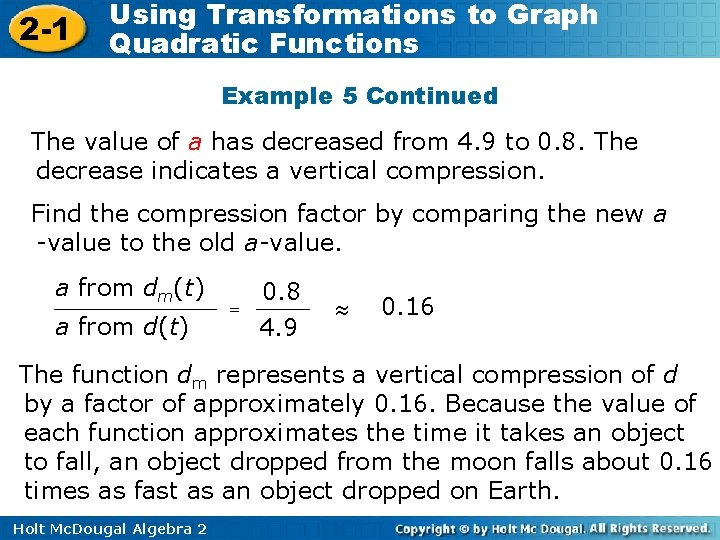2 -1 Using Transformations to Graph Quadratic Functions Example 5 Continued The value of a has decreased from 4. 9 to 0. 8. The decrease indicates a vertical compression. Find the compression factor by comparing the new a -value to the old a-value. a from dm(t) a from d(t) = 0. 8 4. 9 0. 16 The function dm represents a vertical compression of d by a factor of approximately 0. 16. Because the value of each function approximates the time it takes an object to fall, an object dropped from the moon falls about 0. 16 times as fast as an object dropped on Earth. Holt Mc. Dougal Algebra 2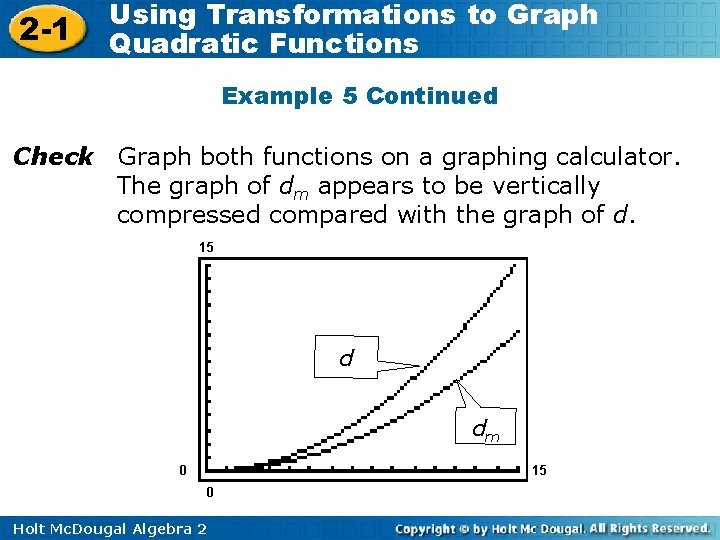2 -1 Using Transformations to Graph Quadratic Functions Example 5 Continued Check Graph both functions on a graphing calculator. The graph of dm appears to be vertically compressed compared with the graph of d. 15 d dm 0 15 0 Holt Mc. Dougal Algebra 2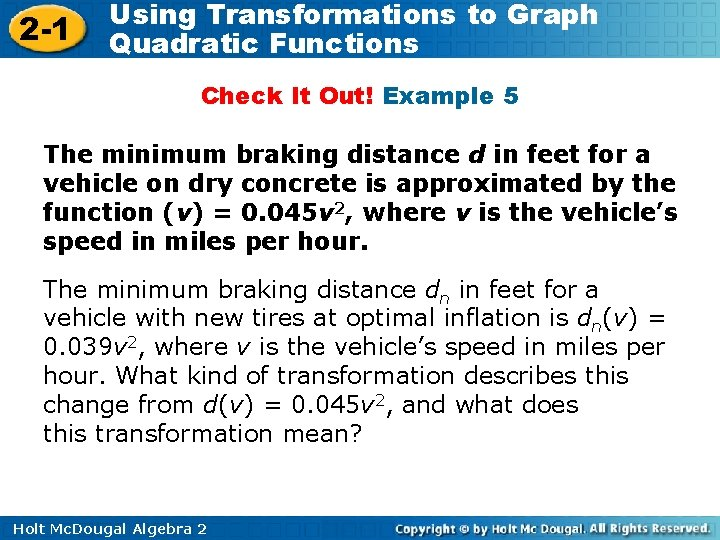2 -1 Using Transformations to Graph Quadratic Functions Check It Out! Example 5 The minimum braking distance d in feet for a vehicle on dry concrete is approximated by the function (v) = 0. 045 v 2, where v is the vehicle’s speed in miles per hour. The minimum braking distance dn in feet for a vehicle with new tires at optimal inflation is dn(v) = 0. 039 v 2, where v is the vehicle’s speed in miles per hour. What kind of transformation describes this change from d(v) = 0. 045 v 2, and what does this transformation mean? Holt Mc. Dougal Algebra 2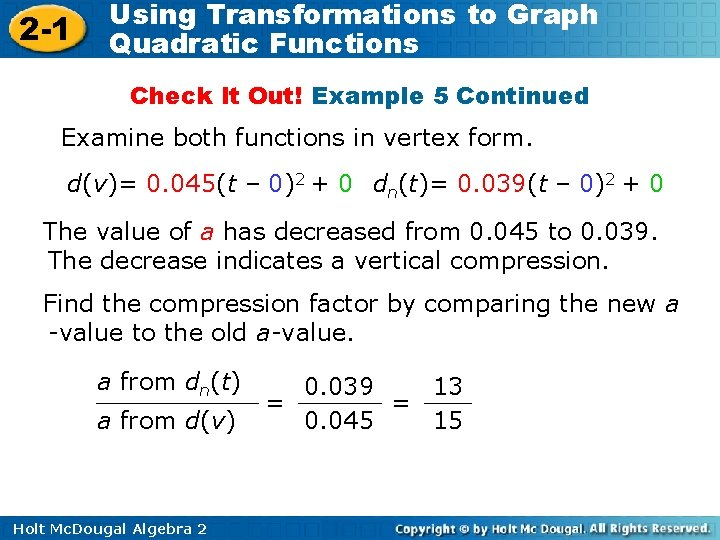2 -1 Using Transformations to Graph Quadratic Functions Check It Out! Example 5 Continued Examine both functions in vertex form. d(v)= 0. 045(t – 0)2 + 0 dn(t)= 0. 039(t – 0)2 + 0 The value of a has decreased from 0. 045 to 0. 039. The decrease indicates a vertical compression. Find the compression factor by comparing the new a -value to the old a-value. a from dn(t) a from d(v) Holt Mc. Dougal Algebra 2 0. 039 13 = = 0. 045 152 -1 Using Transformations to Graph Quadratic Functions Check It Out! Example 5 Continued The function dn represents a vertical compression of d by a factor of. The braking distance will be less with optimally inflated new tires than with tires having more wear. Check Graph both functions on a graphing calculator. The graph of dn appears to be vertically compressed compared with the graph of d. 15 d d n 0 15 0 Holt Mc. Dougal Algebra 2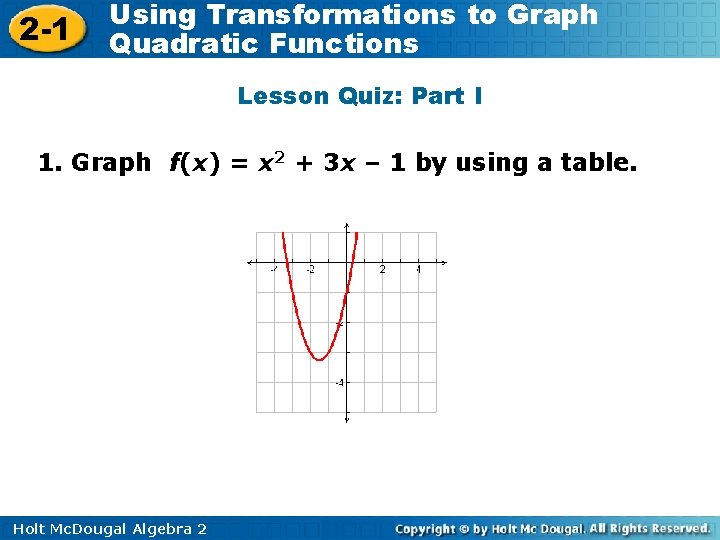2 -1 Using Transformations to Graph Quadratic Functions Lesson Quiz: Part I 1. Graph f(x) = x 2 + 3 x – 1 by using a table. Holt Mc. Dougal Algebra 2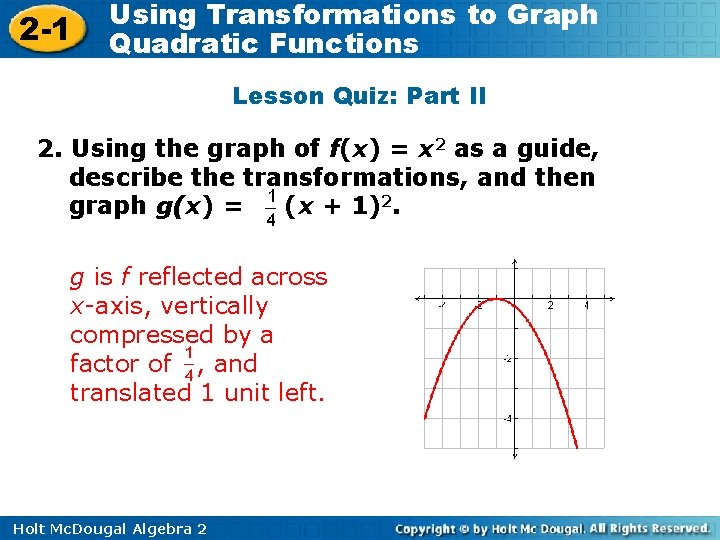2 -1 Using Transformations to Graph Quadratic Functions Lesson Quiz: Part II 2. Using the graph of f(x) = x 2 as a guide, describe the transformations, and then graph g(x) = (x + 1)2. g is f reflected across x-axis, vertically compressed by a factor of , and translated 1 unit left. Holt Mc. Dougal Algebra 22 -1 Using Transformations to Graph Quadratic Functions Lesson Quiz: Part III 3. The parent function f(x) = x 2 is vertically stretched by a factor of 3 and translated 4 units right and 2 units up to create g. Write g in vertex form. g(x) = 3(x – 4)2 + 2 Holt Mc. Dougal Algebra 2